### Exponents and Powers - Revision Notes

CBSE Class–VII Subject Mathematics

Revision Notes
Chapter –13
Exponents and Powers

• Exponents: Exponents are used to express large numbers in shorter form to make them easy to read, understand, compare and operate upon.
• Expressing Large Numbers in the Standard Form: Any number can be expressed as a decimal number between 1.0 and 10.0 (including 1.0) multiplied by a power of 10. Such form of a number is called its standard form or scientific motion.
• Very large numbers are difficult to read, understand, compare and operate upon. To make all these easier, we use exponents, converting many of the large numbers in a shorter form.
• The following are exponential forms of some numbers?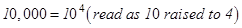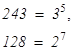.
Here, 10, 3 and 2 are the bases, whereas 4, 5 and 7 are their respective exponents.  We also say, 10,000 is the 4th  power of 10, 243 is the 5th  power of 3, etc.
• Numbers in exponential form obey certain laws, which are: For any non-zero integers a and b and whole numbers m and n,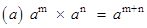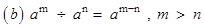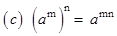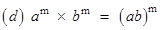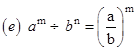(f)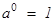(g) (–1) even number = 1 (–1)  odd number = – 1